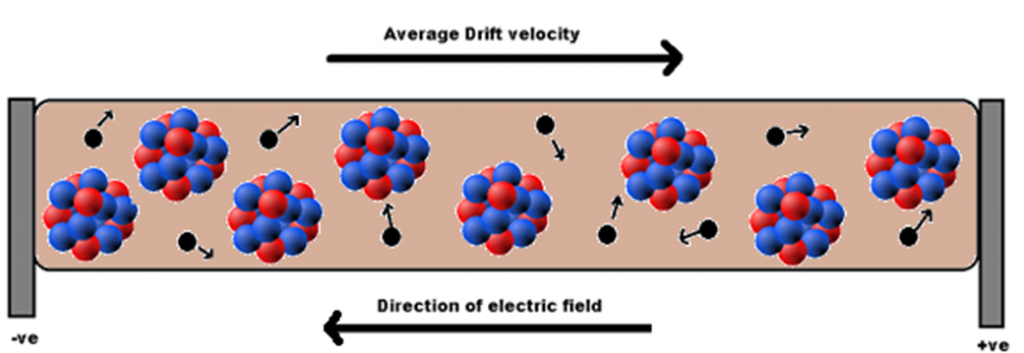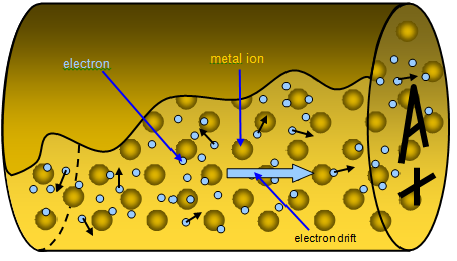Click to Chat

1800-1023-196

+91-120-4616500

CART 0

• 0

MY CART (5)

Use Coupon: CART20 and get 20% off on all online Study Material

ITEM
DETAILS
MRP
DISCOUNT
FINAL PRICE
Total Price: Rs.

There are no items in this cart.
Continue Shopping• Complete Physics Course - Class 11
• OFFERED PRICE: Rs. 2,968
• View Details

```Drift of Electrons and the Origin of Resistivity

Drift of Electrons and the Origin of Resistivity

Drift Velocity and Current

Relation between Drift Velocity, Current Density and Resistivity

Summary

Drift of Electrons and the Origin of Resistivity

We know that under normal circumstances the free electrons move randomly from one atom to another. The velocity with which this random motion is taking place is called Thermal Velocity. The average thermal velocity is said to be zero as the entire motion on an average is random. This is the condition where no electric field is applied.

When an external electric field is applied on the conductor, the electrons or the negative charges are attracted towards the positive charge. When the electrons rush out from the atom, the atom then becomes a positive ion. Thus, these positive ions are attracted towards the negative charge. But as the mass of the positive ion is very high comparing with the electrons, the positive ions do not move. The electrons are accelerated towards the positive charge. A number of collisions also occur with those positive ions and thus these electrons possess a velocity. The average of this velocity is called the drift velocity. With the drift velocity, the flow of electrons is drifted towards the positive end.Drift of electrons

Consider a conductor where the potential difference between the ends is V and L is the length of the conductor.

So E = V / L.

Under the influence of an external electric field, each electron has a force.

The force experienced by each electron, F = - e E

We know that force F = ma, where m is the mass and a is the acceleration.

So ma = - e E.

So when an electric field is applied, each electron has an acceleration which will be opposite to the direction of the field. a = - e E / m. Here e is the charge of an electron, E is the electric field applied and m is the mass of the electron. As the electric field is applied, the electrons will be strongly attracted towards the positive terminal. Thus they moves fast and thus accelerate.Drifting of electrons

The gain in velocity of electrons due to acceleration is lost during the collision with the positive ions. During collision, the positive ions also experiences the force. But due to the heavy mass of positive ions they do not move.

Suppose the velocity of n free electrons at any given instant = u1 + u2 +u3…………………….un.

The vector sum of these velocities = 0

When the electrons are subjected to an electric field and after collision the velocity of the electron is changed.

= v1 + v2 + v3………….vn.

Here v1 = u1 + aԏ1. Here ԏ1 is the time between two successive collisions of the first electron.

Similarly, v2 = u2 + a ԏ2.

Finally, vn = un + a ԏn.

The average velocity of all the free electrons is equal to the drift velocity vd.

vd = v1 + v2 + v3………….vn / n. Substituting the values of v1, v2, v3………….vn, we get

vd = (u1 + aԏ1) + (u2 + a ԏ2) +………………. (un + a ԏn) / n

= (u1 + u2 + ………un / n) + (aԏ1 + a ԏ2 + a ԏn) / n

= u1 + u2 + ………un / n + a (ԏ1 + ԏ2 + ………… ԏn) / n

Thus by simplifying we get vd = u + aԏ, where ԏ = ԏ1 + ԏ2 + ………… ԏn / n.

This average time elapsed between two successive collision of free electrons is called the relaxation time.

As the average velocity = 0

vd = aԏ, as the vector sum of velocities is equal to zero.

We have seen that a = - e E / m. Substitute the value of a in the equation.

So vd = - e E ԏ / m.

Drift velocity is directly proportional to the electric field applied and the average time collapsed between the successive collisions. It is inversely proportional to the mass of the electron.

Drift Velocity and Current

Consider that in a conductor of length L and the area of the conductor is A. Now there are n number of free electrons per unit volume. That is n is the number density of the electrons.Drift of electrons and current

The total number of electrons in the conductor is the product of number density and Volume.

The total number of electrons = n V0, where V0 is the volume.

= n A L

The charge on the total number of electrons in the conductor = e n A L

If the potential difference between the two ends of the conductor is V, then the electric field E = V / L.

As the electric field is applied the electrons are drifted from their position.

The time taken for the electron to reach the other end of the conductor = L / vd

So current I = e n A L / (L / vd) = e n A vd

That is when the electrons move with a drift velocity vd, at a time interval Δt, each electron moves by a distance vd Δt and also the volume of that portion will be A vd Δt. As the number of electrons is n A vd Δt, the charge ΔQ = n e A vd Δt.

So I = ΔQ / Δt = n e A vd Δt / Δt = n e A vd.

Thus it is clear that the current is directly proportional to the drift velocity of the electron. When the drift velocity is increased, the current velocity is also increased.

Substituting the value of vd

We get I = n e2 E A ԏ / m.

Relation between Drift Velocity, Current Density and Resistivity

The current density of a conductor is defined as the current flowing per unit area, where the area is being normal to the current.

Current density J = I / A, I is the current and A is the area.

= n e A vd / A = n e vd

= n e2 ԏ E/ m

The conductivity of the material is given by σ = n e2 ԏ / m.

So, J = σ E

Thus, the resistivity of the material is given by ρ = m / n e2 ԏ

We have seen that vd = - e E ԏ / m

We know that E = - V / L.

Thus vd = e V ԏ / m L , Here e is the charge of the electron, V is the potential difference between the ends of the conductor, ԏ is the relaxation time, m is the mass of the electron and L is the length of the conductor.

From the above expression, we get V = vd m L / e ԏ - equation 1

Also, I = n e A vd. Here A is the area of the cross section and n is the charge density.

So vd = I / n e A.

Substituting this value of vd in equation 1 we get V = (I / n e A) m L / e ԏ = (m L / A n e2 ԏ) I

Re arranging the values, we can write V = (m / n e2 ����) L / A * I

We know that the value (m / n e2 ԏ) is the resistivity of the conductor.

Hence V = ρ L / A * I

The mobility of a moving charge carrier in a conducting material is the magnitude of the drift velocity per unit electric field.

Summary

When an electric field is not applied, no net current is present in any direction due the random thermal velocities of the electrons.

When an electric field is applied, each electron experiences an acceleration which is opposite to the direction of the field. It is given as a = -e E / m. With the drift velocity, the flow of electrons is drifted towards the positive end.

The average drift velocity of the electrons, vd = - e E ԏ / m, if ԏ is the average time between the collisions.

Current density J = - n e vd = n e2 ԏ E/ m, the conductivity of the material is σ = n e2 ԏ / m. So, resistivity ρ = m / n e2 ԏ.

Watch this Video for more reference

Drift of Electrons and the Origin of Resistivity
```### Course Features

• 101 Video Lectures
• Revision Notes
• Previous Year Papers
• Mind Map
• Study Planner
• NCERT Solutions
• Discussion Forum
• Test paper with Video Solution